Do you like the article?
Share it with others -

Use new possibilities of MetaTrader 5

# Effective Averaging Algorithms with Minimal Lag: Use in Indicators

8 February 2007, 09:39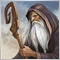3
5 966

### Introduction

I think there is no need to explain how important the smoothing algorithms are for technical analysis and for trading systems. Codes of practically all indicators contain explicit or implicit averaging algorithms. If we have a closer look at online trading platforms and client terminals, the most of them and the most indicators will turn out to use the simplest (though far not the most effective) averaging algorithms.

Much more effective averaging algorithms have been developed by the present. However, attempts to apply them to indicators usually, due to significant complexity of the algorithms, resulted in that the programmers just did not have enough patience and made at most one or two indicators that by no means always operated correctly. After that, they usually tired of working in this direction. The basic advantage of simple averages is that they are always available as simple custom functions to be applied anywhere and at any time.

### Subject Matter

In this article, I would like to describe for traders who know MQL4 rather effective averaging algorithms with minimal lag represented as rather simple custom functions. The use of these functions is not much more complicated than that of technical indicators. The functions were written long before and their operation quality has been checked for rather long time, too. No faults or problems, or incorrect calculations have found in them. Thus, we will consider the following algorithms:
- JJMASeries () - adaptive JMA smoothing algorithm;
- JLiteSeries() - JMA smoothing algorithm without an adaptive algorithm;
- JurXSeries () - ultralinear smoothing algorithm taken from indicator JRSX;
- ParMASeries() - smoothing algorithm based on parabolic approximation;
- LRMASeries () - smoothing algorithm based on linear regression;
- T3Series () - smoothing algorithm based on Tilson algorithm.

### Smoothing Functions Realization

The functions are represented as the following files: JJMASeries.mqh, JLiteSeries. mqh, JurXSeries. mqh, ParMASeries.mqh, LRMASeries.mqh, T3Series.mqh.

Function calls themselves are absolutely the same, the only difference is that some functions do not have some external variables. Such functions are usually used to process custom and indicator arrays that operate as external variables. In my opinion, it is not always convenient, so it would be much better to use such functions for processing normal variables, not arrays. In this case, one can make an unlimited amount of smoothings using these algorithms within one computation cycle! I think it is unnecessary to give the code of functions in this article. The code will be interesting only for those who are going to create similar functions based on other algorithms. We are interested in just the function call algorithm in the indicator code, i.e., in practical use of the functions.

JJMASeries ()
We will start to learn them with function JJMASeries():
```double JJMASeries(int number, int din, int MaxBar, int limit,
int Phase, int Length, double series, int bar,
int& reset)```

File JJMASeries.mqh contains four functions: JJMASeries(), JJMASeriesResize(), JJMASeriesAlert() and JMA_ErrDescr(). The file also contains variables declared as global ones.

Function JJMASeries() is intended for using the JMA algorithm in writing any technical indicators or Expert Advisors, for replacing classical averaging computation with this algorithm. The function does not work if the 'limit' parameter takes on a value of zero! All indicators I developed for JJMASeries are made considering this limitation. The file must be saved in folder MetaTrader\experts\include\. It must be noted that, if the 'bar' variable value exceeds that of the MaxBar variable, the JJMASeries() function will return a zero value for this bar! And, therefore, this value may not be present as a term of a fraction in some indicator calculations! JJMASeries() will return zero on the consequent 30 bars, as well!

This version of JJMASeries() supports Expert Advisors when operated in custom indicators used by the Expert Advisor. Besides, this version of JJMASeries() supports Expert Advisors when operated in the indicator code entirely placed in the Expert's code keeping all the DO statements and variables saved! When coding indicators or Expert Advisors using JJMASeries, it is not recommended to name variables with names starting with nJMA... or dJMA... . The JJMASeries() function can be used in the internal code of other custom functions provided that it is considered that every call for JJMASeries() must have its unique number at each call for such a custom function. This version of JJMASeries() is intended for processing variables related to time-series arrays of the current chart! If this function is applied to processing of variables computed on time-series arrays of other charts, the calculations will be incorrect!

Inputs:
- number - the number of the JJMASeries() function call in the indicator's code (0, 1, 2, 3, ...);
- din - parameter, which allows to modify Length and Phase parameters on each bar. 0 - parameters may not be changed, any other value allows changing parameters;
- MaxBar - maximum value of the computed bar number. Normally, it is Bars-1-period where "period" is the amount of bars, on which the initial series value is not calculated;
- limit - amount of uncalculated bars plus one or the number of the last uncalculated bar. It must be equal to Bars-IndicatorCounted()-1;
- Length - averaging depth;
- Phase - parameter changing in the range between -100 and +100. It influences the transient process quality;
- series - input, which underlies JJMASeries() calculation;
- bar - the number of bar to be calculated. This parameter must be changed by the DO statement from the maximal value to zero. Its maximal value must be always equal to the value of 'limit'!

Output Parameters:
- JMASeries() - JMA value. If the 'bar' parameter value exceeds MaxBar-30, function JJMASeries() always returns zero!
- reset - parameter that returns by reference a value other than 0 if an error occurred in the function computation, and it returns 0 if computation was ok. This parameter can only be variable, but not value!

Function Initialization
Before calling the JJMASeries() function, when the amount of bars already calculated equals to 0, internal buffer variables of the function should be resized (it would be even better to do it in the initialization block of the custom indicator or of the Expert Advisor). For this, it is necessary to call variables of JJMASeries() using helper function JJMASeriesResize() with the following parameters: JJMASeriesResize(number+1); it is necessary to make parameter 'number' (MaxJMA.number) equal to the amount of calls for JJMASeries, i.e., greater by 1 than the maximal value of 'number'. Along with resizing the buffers of JJMASeries(), one can check in the initialization block the indicator input values Length and Phase that are JJMASeries() inputs, for whether they lie within their changing range using JJMASeriesAlert():

`JJMASeriesAlert(int Number, string name, int ExternVar)`

- Number - parameter that can take on two values: 0 - to check the input ExternVar for whether it lies within the changing range of the Length input of JJMASeries() and 1 - to check the input ExternVar for whether it lies within the changing range of the Phase input of JJMASeries();
- name - string name of input ExternVar to give an alert;

- ExternVar - indicator's input

Error Indication

When being debugged, codes of indicators or Expert Advisors can contain errors. To find out the causes of errors, it is necessary to view the log file. Function JJMASeries() records all errors ina log file in the folder named \MetaTrader\EXPERTS\LOGS\. If an MQL4 error occurs in the code preceding JJMASeries() function before calling this function, the function will record the error code and content into a log file. If an MQL4 error occurs in the JJMASeries() algorithm during execution of function JJMASeries(), the function will record the error code and content into a log file, too. If the JJMASeries() function call 'number' is specified incorrectly or incorrect defining of buffer variables size nJJMAResize.Size takes place, messages about incorrect parameters will be recorded in the log file. Information about incorrect definitions of the 'limit' parameter will be recorded in the log file, as well.

If resizing of JJMASeries buffers fails during init() function execution, function JJMASeriesResize() will record information about failed resizing into the log file. If the correct sequence of the 'bar' parameter changing is violated when calling the JJMASeries() function via an external DO statement, this information will be recorded in the log file, too. It must be considered that some errors in the code will produce further errors in its execution, this is why, if the JJMASeries() function records several errors in the log file at once, these errors should be eliminated in order of their appearance. In a correctly coded indicator, the JJMASeries() function can make records in the log file only operating system disorders. An exception is record of buffer variables resizing at indicator or Expert Advisor rebooting that happens at each init() function call. All MQL4 errors are recorded in the log file using function JMA_ErrDescr() that flushes the code and the error content according to its code got using function GetLastError() to the log file.

Exemplary JJMASeries() Function Call (doubled JMA smoothing of the entry price):

```/*
For the indicator to operate, files
JJMASeries.mqh
PriceSeries.mqh
must be placed into the directory: MetaTrader\experts\include\
Heiken Ashi#.mq4
*/
//+------------------------------------------------------------------+
//|                                                        J2JMA.mq4 |
//|                                         http://www.jurikres.com/ |
//|                              Khabarovsk,   farria@mail.redcom.ru |
//+------------------------------------------------------------------+
//---- drawing of the indicator in the main window
#property indicator_chart_window
//---- amount of indicator buffers
#property indicator_buffers 1
//---- color of the indicator
#property indicator_color1 Magenta
//---- INDICATOR INPUTS
extern int Length1 = 5; // depth of the first smoothing
extern int Length2 = 5; // depth of the second smoothing
// the first smoothing parameter changing within the range between -100 and +100,
//it influences the transient quality;
extern int Phase1  = 100;
// the second smoothing parameter changing in the range between -100 and +100,
//it influences the transient quality;
extern int Phase2  = 100;
// indicator shifting along the time axis
extern int Shift   = 0;
/* Choosing of prices to be used for indicator calculations
(0-CLOSE, 1-OPEN, 2-HIGH, 3-LOW, 4-MEDIAN, 5-TYPICAL, 6-WEIGHTED,
7-Heiken Ashi Close, 8-SIMPL, 9-TRENDFOLLOW, 10-0.5*TRENDFOLLOW,
11-Heiken Ashi Low, 12-Heiken Ashi High,  13-Heiken Ashi Open,
14-Heiken Ashi Close.) */
extern int Input_Price_Customs = 0;
//---- indicator buffers
double J2JMA[];
//---- floating points variables
double Temp_Series;
//----+ Introducing of function JJMASeries
//----+ Introducing of function JJMASeriesResize
//----+ Introducing of function JMA_ErrDescr
#include <JJMASeries.mqh>
//----+ Introducing of function PriceSeries
#include <PriceSeries.mqh>
//+------------------------------------------------------------------+
//| J2JMA indicator initialization function                          |
//+------------------------------------------------------------------+
int init()
{
//---- defining of the chart drawing style
SetIndexStyle (0, DRAW_LINE);
//---- 1 indicator buffer is used for calculations
SetIndexBuffer(0, J2JMA);
//---- horizontal shift of the indicator line
SetIndexShift (0, Shift);
//---- placing of indicator values that will not be visible in the chart
SetIndexEmptyValue(0, 0);
//---- name for data windows and label for subwindows
IndicatorShortName ("J2JMA(Length1=" + Length1 + ", Phase1=" + Phase1 +
", Length2=" + Length2 + ", Phase2=" + Phase2 +
", Shift=" + Shift + ")");
SetIndexLabel (0, "J2JMA");
//---- Setting the indicator imaging precision format
IndicatorDigits(Digits);
//----+ Resizing of buffer variables of function JJMASeries,
//nJMAnumber=2(two calls for function JJMASeries)
if(JJMASeriesResize(2) != 2)
return(-1);
//---- setting alerts for nonaccepted values of external variables
//---- complete initialization
return(0);
}
//+------------------------------------------------------------------+
//| J2JMA iteration function                                         |
//+------------------------------------------------------------------+
int start()
{
//---- Bar quantity control over sufficiency for further calculations
if(Bars - 1 < 61)
return(0);
//----+ Introducing of integer variables and obtaining of bars already computed
int reset, MaxBar1, MaxBar2, counted_bars = IndicatorCounted();
//---- checking for possible errors
if(counted_bars < 0)
return(-1);
//---- the last counted bar should be recalculated
//---- (without this recalculation for counted_bars, function JJMASeries will not
//     operate correctly!!!)
if(counted_bars > 0)
counted_bars--;
//---- determining of the oldest bar number, starting from which new bars
//     will be recalculated
int limit = Bars - counted_bars - 1;
MaxBar1 = Bars - 1;
MaxBar2 = MaxBar1 - 30;

//----+ INDICATOR COMPUTING BASIC LOOP
for(int bar = limit; bar >= 0; bar--)
{
// Call for function PriceSeries to get the entry price Series
Temp_Series = PriceSeries(Input_Price_Customs, bar);
// Two calls fro function JJMASeries numbered as 0,1. Parameters
//nJMA.Phase and nJMA.Length
//do not change at each bar (nJMA.din=0)
//(In the second call, parameter nJMA.MaxBar is decreased by 30 since it is
//the repeated JMA smoothing)
Temp_Series = JJMASeries(0,0,MaxBar1,limit,Phase1,Length1,
Temp_Series,bar,reset);
// checking for errors in the preceding operation
if(reset != 0)
return(-1);
Temp_Series = JJMASeries(1,0,MaxBar2,limit,Phase2,Length2,
Temp_Series,bar,reset);
// checking for errors in the preceding operation
if(reset != 0)
return(-1);
J2JMA[bar] = Temp_Series;
}
//---- complete calculation of indicator values
return(0);
}
//+--------------------------------------------------------+```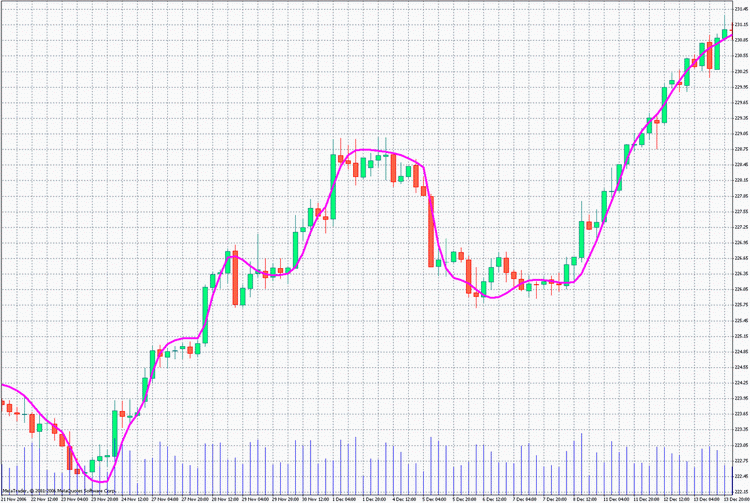Thus, the following points can be stressed in application of this function:
1. Declaration of functions being parts of file JJMASeries.mqh with line #include in the beginning of the indicator code. Declared are variables and four functions: JJMASeries(), JJMASeriesResize(), JJMASeriesAlert(), JMA_ErrDescr().
2. Resizing of buffer elements used by function JJMASeries() using function JJMASeriesResize() in the initialization block.
3. Checking using function JJMASeriesAlert() in the initialization block whether the values of the indicator external variables that are external variables of function JJMASeries() are correct .
4. Calls for function JJMASeries() themselves made using DO statements with relevant error control. >

### Other Functions

Algorithm of calling for other functions is very much the same as the algoritm considered above, but there are some differences in quantity of external variables available in functions:
```JJMASeries (int number, int din, int MaxBar, int limit, int Phase,
int Length, double series, int bar, int&reset)
JLiteSeries(int number, int din, int MaxBar, int limit, int Phase,
int Length, double series, int bar, int&reset)
JurXSeries (int number, int din, int MaxBar, int limit,
int Length, double series, int bar, int&reset)
T3Series   (int number, int din, int MaxBar, int limit, int Phase,
int Length, double series, int bar, int&reset )
ParMASeries(int number, int MaxBar, int limit, int period,
double series, int bar, int&reset)
LRMASeries (int number, int MaxBar, int limit, int period,
double series, int bar, int&reset )```

It should be appreciated that functions JJMASeries() and JLiteSeries() are not compatible in the same Expert Advisor or indicator! Indeed, the same JMA code with the function name of JJMASeries()is placed in file JLiteSeries.mqh without adaptation! To replace function JJMASeries() with function JLiteSeries() in an Expert Advisor or in an indicator, it is sufficient to replace line #include with #include. All calls for functions of file JLiteSeries.mqh are considered as calls for functions identical to those used for functions of file JJMASeries.mqh.

Other functions are fully compatible within the same indicator or Expert Advisor code. In functions ParMASeries() and LRMASeries(), the value of external variable 'period' is limited by 501. If larger values are necessary, it is necessary to change the first (not zero!) parameters of buffers dParMA.TempBuffer[] and dParMA.TEMPBUFFER[] for function ParMASeries() or dLRMA.TempBuffer[] and dLRMA.TEMPBUFFER[] for function LRMASeries() in files ParMASeries.mqh and LRMASeries.mqh, respectively. >

Function JurXSeries()
Below is an exemplary call for function JurXSeries() (ultralinear smoothing of the entry price with additional JMA smoothing):

```/*
For the indicator to operate, it is necessary to place files

JurXSeries.mqh,
JJMASeries.mqh,
PriceSeries.mqh,
Heiken Ashi#.mq4
This indicator is based on the smoothing algorithm of indicator JRSX.
The final result of this indicator bear some resemblance to
double JMA smoothing, but is less perfect since it is simpler.

*/
//+------------------------------------------------------------------+
//|                                                        JJurX.mq4 |
//|                              Khabarovsk,   farria@mail.redcom.ru |
//+------------------------------------------------------------------+
//---- drawing the indicator in the main window
#property indicator_chart_window
//---- amount of indicator buffers
#property indicator_buffers 1
//---- color of the indicator
#property indicator_color1 Gold
//---- INDICATOR INPUTS
extern int JurX_Length  = 5; // depth of JurX smoothing
extern int JJMA_Length  = 4; // depth of JJMA smoothing
// parameter of JJMA smoothing ranging between -100 and +100
// influences the transient quality;
extern int JJMA_Phase   = -100;
extern int Shift        = 0;      // indicator shift along the time axis
/* Choosing of prices underlying the indicator calculations
(0-CLOSE, 1-OPEN, 2-HIGH, 3-LOW, 4-MEDIAN, 5-TYPICAL, 6-WEIGHTED,
7-Heiken Ashi Close, 8-SIMPL, 9-TRENDFOLLOW, 10-0.5*TRENDFOLLOW,
11-Heiken Ashi Low, 12-Heiken Ashi High,  13-Heiken Ashi Open,
14-Heiken Ashi Close.) */
extern int Input_Price_Customs = 0;
//---- indicator buffers
double Ind_Buffer[];
//---- floating point variables
double Price,JurX,JJurX,Error;
//+------------------------------------------------------------------+
//----+ Introducing of function JJMASeries
//----+ Introducing of function JJMASeriesResize
//----+ Introducing of function JMA_ErrDescr
#include <JJMASeries.mqh>
//+------------------------------------------------------------------+
//----+ Introducing of function JurXSeries
//----+ Introducing of function JurXSeriesResize
//----+ Introducing of function JurX_ErrDescr
#include <JurXSeries.mqh>
//+------------------------------------------------------------------+
//----+ Introducing of function PriceSeries
#include <PriceSeries.mqh>
//+------------------------------------------------------------------+
//| JJurX indicator initialization function                          |
//+------------------------------------------------------------------+
int init()
{
//---- defining of the charting style
SetIndexStyle (0,DRAW_LINE);
//---- 1 indicator buffer is used to calculate
SetIndexBuffer(0,Ind_Buffer);
//---- horizontal shift of the indicator line
SetIndexShift (0, Shift);
//---- setting indicator values that will not be visible in the chart
SetIndexEmptyValue(0,0);
//---- name for data windows and label for subwindows
IndicatorShortName ("JJurX( JurX_Length="+JurX_Length+", Shift="+Shift+")");
SetIndexLabel (0, "JJurX");
//---- Setting the indicator imaging precision format
IndicatorDigits(Digits);
//----+ Resizing buffer variables of function JurXSeries,
//      nJurXnumber=2
//(To calls for function JurXSeries)
if (JurXSeriesResize(2)!=2)return(-1);
//----+ Resizing buffer variables of function JJMASeries,
//      nJMAnumber=1
//(One call for function JJMASeries)
if (JJMASeriesResize(1)!=1)return(-1);
//---- setting alerts for nonaccepted values of external variables
//---- complete initialization
return(0);
}
//+-----------------------------------------------------------------------------+
//| JJurX iteration function                                                    |
//+-----------------------------------------------------------------------------+
int start()
{
//---- Bar quantity control over sufficiency for further calculations
if (Bars-1<JurX_Length+32)return(0);
//----+ Introducing of integer variables and obtaining of bars already computed
int reset,MaxBar,counted_bars=IndicatorCounted();
//---- checking for possible errors
if (counted_bars<0)return(-1);
//---- the last counted bar should be recalculated
//(without this recalculation for counted_bars, function JurXSeries will not
// operate correctly!!!)
if (counted_bars>0) counted_bars--;
//---- determining of the oldest bar number, starting from which new bars
//     will be recalculated
int limit=Bars-counted_bars-1;
determining of the oldest bar number, starting from which all bars
//     will be recalculated
MaxBar=Bars-1;

//----+ INDICATOR COMPUTING BASIC LOOP
for(int bar=limit;bar>=0;bar--)
{
//----+ Call for function PriceSeries to get the entry
//      price Series
Price=PriceSeries(Input_Price_Customs,bar);
//----+ One call for function JurXSeries numbered as 0.
//Parameter nJJurX.Length does not change on each bar (nJurXdin=0)
JurX=JurXSeries(0,0,MaxBar,limit,JurX_Length,Price,bar,reset);
//----+ checking for errors in the preceding operation
if(reset!=0)return(-1);
//----+ detection of error in calculations of parameter JurX
//----+ the second call for function JurXSeries numbered as 1.
//Parameter nJJurX.Length does not change on each bar (nJurXdin=0)
Error=JurXSeries(1,0,MaxBar,limit,JurX_Length,100,bar,reset);
//----+ checking for errors in the preceding operation
if(reset!=0)return(-1);
if(Error==0)Error=100;
JurX*=100/Error;
//----+ Call for function JJMASeries numbered as 0.
//      Parameters nJMA.Phase and nJMA.Length do not change on each bar
//      (nJMA.din=0)
JJurX=JJMASeries(0,0,MaxBar,limit,JJMA_Phase,JJMA_Length,JurX,bar,reset);
//----+ checking for errors in the preceding operation
if(reset!=0)return(-1);
Ind_Buffer[bar]=JJurX;
}
//---- complete calculation of indicator values
return(0);
}
//+-------------------------------------------------------------------------+```

In this example, it should be noted that function JurXSeries() averages both entry price and the constant! Having divided the averaging result by the constant value, we will obtain the smoothing error. To obtain a more precise results of the price series smoothing, it is necessary to divide the smoothing result by this error value. It was done, in our case. In two cases below, numerator and denominator are smoothed separately, so there is no need of the above procedure. Such error does not occur for other smoothing functions.

Below is an exemplary call for functions JJMASeries() and JurXSeries() (CCI analog with additional JMA smoothing):

```/*
For the indicator to operate, it is necessary to place files
JJMASeries.mqh
JurSeries.mqh
PriceSeries.mqh
Heiken Ashi#.mq4
*/
//+------------------------------------------------------------------+
//|                                                        JCCIX.mq4 |
//|                              Khabarovsk,   farria@mail.redcom.ru |
//+------------------------------------------------------------------+
//---- drawing the indicator in a separate window
#property indicator_separate_window
//---- amount of indicator buffers
#property indicator_buffers  1
//---- colors of indicator
#property indicator_color1  BlueViolet
//---- parameters of the indicator horizontal levels
#property indicator_level1  0.5
#property indicator_level2 -0.5
#property indicator_level3  0.0
#property indicator_levelcolor MediumBlue
#property indicator_levelstyle 4
//---- INDICATOR INPUTS
extern int  JJMA.Length = 8;  // depth of JJMA smoothing of entry price
// depth of JurX smoothing of the obtained indicator
extern int  JurX.Length = 8;
// parameter ranging between -100 and +100 influences
// the smoothing transient quality
extern int  JJMA.Phase = 100;
/* Choosing of prices underlying the indicator calculations
(0-CLOSE, 1-OPEN, 2-HIGH, 3-LOW, 4-MEDIAN, 5-TYPICAL, 6-WEIGHTED,
7-Heiken Ashi Close, 8-SIMPL, 9-TRENDFOLLOW, 10-0.5*TRENDFOLLOW,
11-Heiken Ashi Low, 12-Heiken Ashi High,  13-Heiken Ashi Open,
14-Heiken Ashi Close.) */
extern int Input_Price_Customs = 0;
//---- indicator buffers
double Ind_Buffer1[];
//---- integer constans
int    w;
//+------------------------------------------------------------------+
//----+ Introducing of function JJMASeries
//----+ Introducing of function JJMASeriesResize
//----+ Introducing of function JMA_ErrDescr
#include <JJMASeries.mqh>
//+------------------------------------------------------------------+
//----+ Introducing of function JurXSeries
//----+ Introducing of function JurXSeriesResize
//----+ Introducing of function JurX_ErrDescr
#include <JurXSeries.mqh>
//+------------------------------------------------------------------+
//----+ Introducing of function PriceSeries
#include <PriceSeries.mqh>
//+------------------------------------------------------------------+
//| JCCIX initialization function                                    |
//+------------------------------------------------------------------+
int init()
{
//---- indicator drawing styles
SetIndexStyle(0,DRAW_LINE);
//---- 1 indicator buffer is used for calculations.
SetIndexBuffer(0,Ind_Buffer1);
//---- setting of the indicator values that will not be visible in the chart
SetIndexEmptyValue(0,0);
//---- names for data windows and labels for subwindows
SetIndexLabel(0,"JCCIX");
IndicatorShortName("JCCIX(JJMA.Length="+JJMA.Length+", JurX.Length"+
JurX.Length+")");
//---- Setting imaging precision format (count of characters after decimal point)
//to visualize the indicator values
IndicatorDigits(2);
//----+ Resizing buffer variables of function JurXSeries,
//      nJurXnumber=2
//(Two calls for function JurXSeries)
if (JurXSeriesResize(2)!=2)return(-1);
//----+ Resizing buffer variables of function JJMASeries,
//      nJMAnumber=1
//(One call for function JJMASeries)
if (JJMASeriesResize(1)!=1)return(-1);
//---- setting alerts for nonaccepted values of external variables
//---- setting the bar number, starting from which the indicator will be
//     drawn
SetIndexDrawBegin(0,JurX.Length+31);
//---- coefficients initialization to compute the indicator
if (JurX.Length>5) w=JurX.Length-1; else w=5;
//---- initialization complete
return(0);
}
//+------------------------------------------------------------------------+
//|  JCommodity Channel IndexX                                             |
//+------------------------------------------------------------------------+
int start()
{
//---- Introducing of floating point variables
double price,Jprice,JCCIX,UPCCI,DNCCI,JUPCCIX,JDNCCIX;
//----+ Introducing of integer variables and getting bars already computed
int reset,MaxBar,MaxBarJ,limit,counted_bars=IndicatorCounted();
//---- check for possible errors
if (counted_bars<0)return(-1);
//---- the last counted bar must be recalculated
//---- (without this recalculation for counted_bars, functions JJMASeries
//and JurXSeries will not work correctly!!!)
if (counted_bars>0) counted_bars--;
//---- determining of the oldest bar number, starting from which new bars
//     will be recalculated
limit=Bars-counted_bars-1;
//---- determining of the oldest bar number, starting from which all bars
//     will be recalculated
MaxBar=Bars-1; MaxBarJ=MaxBar-30;
//---- correction of the start calculated bar in the loop
if(limit>=MaxBar)limit=MaxBar;

for(int bar=limit; bar>=0; bar--)
{
//----+ Call for function PriceSeries to get entry
//      price Series
price=PriceSeries(Input_Price_Customs, bar);
//+----------------------------------------------------------------
//----+ One call for function JJMASeries numbered as 0
//----+ Parameters nJMA.Phase and nJMA.Length do not change within
//      each bar (nJMA.din=0)
//+---------------------------------------------------------------+
Jprice=JJMASeries(0,0,MaxBar,limit,JJMA.Phase,JJMA.Length,price,
bar,reset);
//----+ check for errors in the preceding operation
if(reset!=0)return(-1);
//+---------------------------------------------------------------+
UPCCI=price-Jprice;
DNCCI=MathAbs(UPCCI);
//----+ Two calls for function JurXSeries numbered as 0 and 1.
Parameter nJJurXLength does not
//change within each bar (nJurXdin=0)
//----+ check for errors in the preceding operation
JUPCCIX=JurXSeries(0,0,MaxBarJ,limit,JurX.Length,UPCCI,bar,reset);
if(reset!=0)return(-1);
JDNCCIX=JurXSeries(1,0,MaxBarJ,limit,JurX.Length,DNCCI,bar,reset);
if(reset!=0)return(-1);
//----+
if (bar>MaxBarJ-w)JCCIX=0;
else
if (JDNCCIX!=0)
{
JCCIX=JUPCCIX/JDNCCIX;
if(JCCIX>1)JCCIX=1;
if(JCCIX<-1)JCCIX=-1;
}
else JCCIX=0;
Ind_Buffer1[bar]=JCCIX;
//----+
}
//----
return(0);
}
//+-------------------------------------------------------------------+```

The following fact should be taken into consideration: After two smoothings with function JurXSeries(), one of values obtained will be checked for it is not equal to zero for it is a denominator!

Below is an exemplary call for functions JJMASeries() and JurXSeries (RSI analog with additional JMA smoothing):

```/*
For the indicator to operate, it is necessary to place files
JurXSeries.mqh
JJMASeries.mqh
PriceSeries.mqh
Heiken Ashi#.mq4
*/
//+------------------------------------------------------------------+
//|                                                        JJRSX.mq4 |
//|                              Khabarovsk,   farria@mail.redcom.ru |
//+------------------------------------------------------------------+
//---- drawing of the indicator in a separate window
#property indicator_separate_window
//---- amount of indicator buffers
#property indicator_buffers  1
//---- colors of the indicator
#property indicator_color1  BlueViolet
//---- parameters of the indicator horizontal levels
#property indicator_level1  0.5
#property indicator_level2 -0.5
#property indicator_level3  0.0
#property indicator_levelcolor MediumBlue
#property indicator_levelstyle 4
//---- INDICATOR INPUTS
extern int  Length = 8;  // depth of JurX smoothing of the indicator
// depth of JJMA smoothing of the obtained indicator
extern int  Smooth = 3;
// parameter ranging between -100 and +100, influences
//the smoothing transient quality
extern int  Phase = 100;
/* Choosing of prices, at which the indicator is computed
(0-CLOSE, 1-OPEN, 2-HIGH, 3-LOW, 4-MEDIAN, 5-TYPICAL, 6-WEIGHTED,
7-Heiken Ashi Close, 8-SIMPL, 9-TRENDFOLLOW, 10-0.5*TRENDFOLLOW,
11-Heiken Ashi Low, 12-Heiken Ashi High,  13-Heiken Ashi Open,
14-Heiken Ashi Close.) */
extern int Input_Price_Customs = 0;
//---- indicator buffers
double Ind_Buffer[];
//---- integer variables
int    w;
//+------------------------------------------------------------------+
//----+ Introducing of function JJMASeries
//----+ Introducing of function JJMASeriesResize
//----+ Introducing of function JMA_ErrDescr
#include <JJMASeries.mqh>
//+------------------------------------------------------------------+
//----+ Introducing of function JurXSeries
//----+ Introducing of function JurXSeriesResize
//----+ Introducing of function JurX_ErrDescr
#include <JurXSeries.mqh>
//+------------------------------------------------------------------+
//----+ Introducing of function PriceSeries
#include <PriceSeries.mqh>
//+---------------------------------------------------------------------+
//| JJRSX initialization function                                       |
//+---------------------------------------------------------------------+
int init()
{
//---- indicator drawing styles
SetIndexStyle(0,DRAW_LINE);
//---- 1 indicator buffer is used for counting.
SetIndexBuffer(0,Ind_Buffer);
//---- setting the indicator values that will not be visible in the chart
SetIndexEmptyValue(0,0);
//---- names for data windows and labels for subwindows
SetIndexLabel(0,"JRSX");
IndicatorShortName("JRSX(Length="+Length+", Input_Price_Customs="+
Input_Price_Customs+")");
//---- Setting imaging precision format (count of characters after decimal point)
//to visualize the indicator values
IndicatorDigits(2);
//----+ Resizing buffer variables of function JurXSeries,
nJurXnumber=2
//(Two calls for function JurXSeries)
if (JurXSeriesResize(2)!=2)return(-1);
//----+ Resizing buffer variables of function JJMASeries,
nJMAnumber=1
//(One call for function JJMASeries)
if (JJMASeriesResize(1)!=1)return(-1);
//---- setting alerts for nonaccepted values of external variables
//---- setting the bar number, starting from which there will be drawn the
indicator
SetIndexDrawBegin(0,Length+31);
//---- correction of nonaccepted value ща parameter Length
if(Length<1)Length=1;
//---- coefficients initialization to compute the indicator
if (Length>5) w=Length-1; else w=5;
//---- initialization complete
return(0);
}
//+-----------------------------------------------------------------------------+
//| JJRSX iteration function                                                    |
//+-----------------------------------------------------------------------------+
int start()
{
//---- Introducing floating point variables
double dPrice,dPriceA,UPJRSX,DNJRSX,JRSX,JJRSX;
//----+ Introducing of integer variables and obtaining of bars already computed
int bar,limit,reset,MaxBar,MaxBarJ,counted_bars=IndicatorCounted();
//---- check for possible errors
if (counted_bars<0)return(-1);
//---- the last counted bar must be recalculated
if (counted_bars>0) counted_bars--;
//---- determining of the oldest bar number, starting from which all bars
//     will be recalculated
MaxBar=Bars-2; MaxBarJ=MaxBarJ-w-1;
//---- determining of the oldest bar number, starting from which new bars
//     will be recalculated
limit=Bars-counted_bars-1;
//----+
if (limit>MaxBar){limit=MaxBar;Ind_Buffer[MaxBar]=0.0;}

for(bar=limit;bar>=0;bar--)
{
//----+ two calls for function PriceSeries to get the difference
//      between entry prices dPrice
dPrice = PriceSeries(Input_Price_Customs, bar)-
PriceSeries(Input_Price_Customs, bar+1);
//----+
dPriceA=MathAbs(dPrice);
//----+ Two calls for function JurXSeries numbered as 0 and 1.
//      Parameter nJJurXLength
//does not change on each bar (nJurXdin=0)
//check for errors in the preceding operation
UPJRSX=JurXSeries(0,0,MaxBar,limit,Length,dPrice, bar,reset);
if(reset!=0)return(-1);
DNJRSX=JurXSeries(1,0,MaxBar,limit,Length,dPriceA,bar,reset);
if(reset!=0)return(-1);
//----+
if (bar>MaxBar-w)JRSX=0;
else if (DNJRSX!=0){JRSX=UPJRSX/DNJRSX;
if(JRSX>1)JRSX=1;
if(JRSX<-1)JRSX=-1;}else JRSX=0;
//+---------------------------------------------------------------+
//----+ One call for function JJMASeries numbered as 0
//----+ Parameters nJMA.Phase and nJMA.Length do not change
//      on each bar (nJMA.din=0)
//+---------------------------------------------------------------+
JJRSX=JJMASeries(0,0,MaxBarJ,limit,Phase,Smooth,JRSX,bar,reset);
//----+ check for errors in the preceding operation
if(reset!=0)return(-1);
//+---------------------------------------------------------------+
Ind_Buffer[bar]=JJRSX;
}
//---- complete calculation of the indicator values
return(0);
}
//+------------------------------------------------------------------+```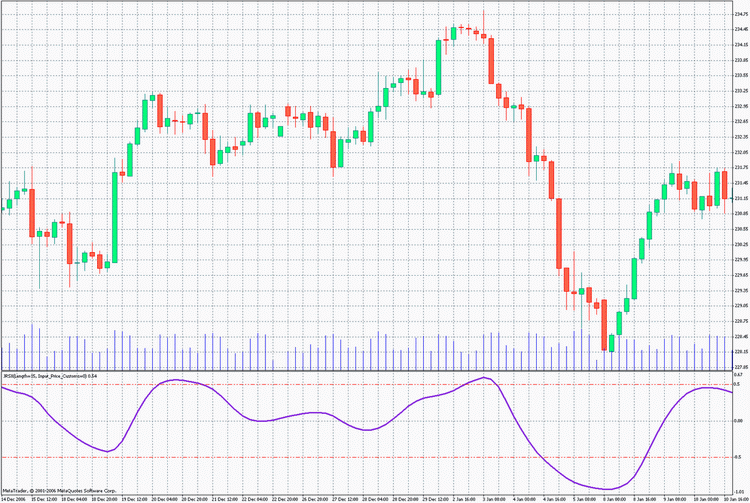Functions T3Series()

Below is an exemplary call for function T3Series() (Three Bollinger Bands with additional T3 smoothing):

```/*
To work with the indicator, files
T3Series.mqh
PriceSeries.mqh
must be placed in directory: MetaTrader\experts\include\
Heiken Ashi#.mq4
*/
//+------------------------------------------------------------------+
//|                                          T3.6Bollinger Bands.mq4 |
//|                              Khabarovsk,   farria@mail.redcom.ru |
//+------------------------------------------------------------------+
//---- drawing of the indicator in the main window
#property indicator_chart_window
//---- amount of indicator buffers
#property indicator_buffers 7
//---- indicator color
#property indicator_color1 Gray
#property indicator_color2 Red
#property indicator_color3 Blue
#property indicator_color4 Lime
#property indicator_color5 Blue
#property indicator_color6 Red
#property indicator_color7 Gray
//---- indicator line style
#property indicator_style1 4
#property indicator_style2 2
#property indicator_style3 4
#property indicator_style4 4
#property indicator_style5 4
#property indicator_style6 2
#property indicator_style7 4
//---- INDICATOR INPUTS
// averaging period of J2Bollinger Bands
extern int        Bands_Period = 100;
extern double Bands_Deviations = 2.0; // deviation
extern int         MA_method = 0;   // averaging method
// smoothing depth of the obtained Moving Avereges
extern int         MA_Smooth = 20;
// smoothing depth of the obtained Bollinger Bands
extern int        Bands_Smooth = 20;
// smoothing parameter ranging between -100 and +100,
// influences the transient quality;
extern int    Smooth_Curvature = 100;
// indicator shift along the time axis
extern int         Bands_Shift = 0;
//Choosing of prices, on which the indicator is calculated
/*(0-CLOSE, 1-OPEN, 2-HIGH, 3-LOW, 4-MEDIAN, 5-TYPICAL, 6-WEIGHTED,
7-Heiken Ashi Close, 8-SIMPL, 9-TRENDFOLLOW, 10-0.5*TRENDFOLLOW,
11-Heiken Ashi High, 12-Heiken Ashi Low, 13-Heiken Ashi Open,
14-Heiken Ashi Close.)*/
extern int Input_Price_Customs = 0;
//---- indicator buffers
double UpperBuffer3  [];
double UpperBuffer2  [];
double UpperBuffer1  [];
double T3MovingBuffer[];
double LowerBuffer1  [];
double LowerBuffer2  [];
double LowerBuffer3  [];
double Series_buffer [];
//+------------------------------------------------------------------+
//----+ Introducing of function T3Series
//----+ Introducing of function T3SeriesResize
//----+ Introducing of function T3_ErrDescr
#include <T3Series.mqh>
//+------------------------------------------------------------------+
//----+ Introducing of function PriceSeries
#include <PriceSeries.mqh>
//+------------------------------------------------------------------+
//| T3.6Bollinger Bands initialization function                      |
//+------------------------------------------------------------------+
int init()
{
//---- defining the chart drawing style
SetIndexStyle(0,DRAW_LINE);
SetIndexStyle(1,DRAW_LINE);
SetIndexStyle(2,DRAW_LINE);
SetIndexStyle(3,DRAW_LINE);
SetIndexStyle(4,DRAW_LINE);
SetIndexStyle(5,DRAW_LINE);
SetIndexStyle(6,DRAW_LINE);
//---- 4 indicator buffers are used for calculations
IndicatorBuffers(8);
SetIndexBuffer(0,UpperBuffer3 );
SetIndexBuffer(1,UpperBuffer2 );
SetIndexBuffer(2,UpperBuffer1 );
SetIndexBuffer(3,T3MovingBuffer);
SetIndexBuffer(4,LowerBuffer1 );
SetIndexBuffer(5,LowerBuffer2 );
SetIndexBuffer(6,LowerBuffer3 );
SetIndexBuffer(7,Series_buffer);
//---- setting up indicator values that will not be visible in the chart
SetIndexEmptyValue(0,0);
SetIndexEmptyValue(1,0);
SetIndexEmptyValue(2,0);
SetIndexEmptyValue(3,0);
SetIndexEmptyValue(4,0);
SetIndexEmptyValue(5,0);
SetIndexEmptyValue(6,0);
//---- setting up the bar number, starting from which the indicator
//     will be drawn
int drawbegin=100+Bands_Shift;
SetIndexDrawBegin(0,drawbegin);
SetIndexDrawBegin(1,drawbegin);
SetIndexDrawBegin(2,drawbegin);
SetIndexDrawBegin(3,drawbegin);
SetIndexDrawBegin(4,drawbegin);
SetIndexDrawBegin(5,drawbegin);
SetIndexDrawBegin(6,drawbegin);
//---- horizontal shift of the indicator lines
SetIndexShift (0, Bands_Shift);
SetIndexShift (1, Bands_Shift);
SetIndexShift (2, Bands_Shift);
SetIndexShift (3, Bands_Shift);
SetIndexShift (4, Bands_Shift);
SetIndexShift (5, Bands_Shift);
SetIndexShift (6, Bands_Shift);
//---- name for data windows and labels for subwindows
IndicatorShortName ("T3.4Bollinger Bands( Period="+Bands_Period+
", Deviations="+Bands_Deviations+")");
SetIndexLabel (0, "Upper3 Bands");
SetIndexLabel (1, "Upper2 Bands");
SetIndexLabel (2, "Upper1 Bands");
SetIndexLabel (4, "Lower1 Bands");
SetIndexLabel (5, "Lower2 Bands");
SetIndexLabel (6, "Lower3 Bands");
string Moving;
switch(MA_method)
{
case  0: Moving= "T3SMA";break;
case  1: Moving= "T3EMA";break;
case  2: Moving="T3SSMA";break;
case  3: Moving="T3LWMA";break;
default: Moving="T3SMA";
}
SetIndexLabel (3, "Moving Avereges "+Moving+" ("+Bands_Period+")");
//---- Setting imaging precision format for the indicator
IndicatorDigits(Digits);
//----+ Resizing of buffer variables of function T3Series,
//nT3.number=7(Seven calls for function T3Series)
if (Bands_Smooth<=1){if (T3SeriesResize(1)!=1)return(-1);}
else if (T3SeriesResize(7)!=7)return(-1);
//---- setting alerts for nonaccepted values of external variables
if((MA_method<0)||(MA_method>3))
Alert("Parameter MA_method must range between 0 and 3"
+ " You input a nonaccepted "
+MA_method+ "0 will be used");
//---- correction of the nonaccepted value of parameter Bands_Period
if(Bands_Period<1)Bands_Period=1;
//---- initialization complete
return(0);
}
//+------------------------------------------------------------------------+
//| T3.6Bollinger Bands iteration function                                 |
//+------------------------------------------------------------------------+
int start()
{
//---- check for whether the amount of bars is sufficient for calculations
if(Bars-1<=Bands_Period) return(0);
//---- Introducing of floating point variables
double deviation1,deviation2,deviation3,Temp_Series,sum,midline,
priceswing,Resalt;
//----+ Introducing of integer variables and getting the bars already calculated
int reset,MaxBar,MaxBarBB,MaxBarBB1,bar,kk,counted_bars=IndicatorCounted();
//---- check for possible errors
if (counted_bars<0)return(-1);
//---- the last counted bar must be recalculated
// (without this recalculation for counted_bars, function T3Series will not work
// correctly!!!)
if (counted_bars>0) counted_bars--;
//---- determining of the oldest bar number, starting from which new bars
//     will be recalculated
int limit=Bars-counted_bars-1;
//---- determining of the oldest bar number, starting from which all bars
//     will be recalculated
MaxBar=Bars-1-Bands_Period; MaxBarBB=MaxBar-30-Bands_Period;
MaxBarBB1=MaxBarBB-1;
for(bar=limit;bar>=0;bar--)
Series_buffer[bar]=PriceSeries(Input_Price_Customs,bar);
//---- checking whether the bars are sufficient for calculation of Bollinger Bands
//---- zero initialization
if (limit>MaxBar)
{
for(bar=limit;bar>=MaxBar;bar--)T3MovingBuffer[bar]=0;
limit=MaxBar;
}
//----+ Moving Averages calculation loop
for(bar=limit;bar>=0;bar--)
{
//----+ Moving Averages calculation formula
Temp_Series=iMAOnArray(Series_buffer,0,Bands_Period,0,
MA_method, bar);
//----+ smoothing of the obtained Moving Averages
//----+ call for function T3Series numbered as 0.
// Parameters nT3.Curvature and nT3.Length do not change on
// each bar (nT3.din=0)
Resalt=T3Series(0,0,MaxBar,limit,Smooth_Curvature,MA_Smooth,
Temp_Series,bar,reset);
//----+ check for error in the preceding operation
if(reset!=0)return(-1);
T3MovingBuffer[bar]=Resalt;
}
//---- CALCULATION of Bollinger Bands
//---- zero initialization
if (limit>MaxBarBB)
{
for(bar=limit;bar>=MaxBarBB;bar--)
{
UpperBuffer2[bar]=0;
UpperBuffer1[bar]=0;
LowerBuffer1[bar]=0;
LowerBuffer2[bar]=0;
}
limit=MaxBarBB;
}
for(bar=limit;bar>=0;bar--)
{
sum=0.0;
midline=T3MovingBuffer[bar];
kk=bar+Bands_Period-1;
while(kk>=bar)
{
priceswing=PriceSeries(Input_Price_Customs,kk)-midline;
sum+=priceswing*priceswing;
kk--;
}
deviation2=Bands_Deviations*MathSqrt(sum/Bands_Period);
deviation1=0.5*deviation2;
deviation3=1.5*deviation2;
if (Bands_Smooth>1)
{
//----+ calculation and T3 smoothing of Bollinger Bands
//----+ ------------------------------------------------------+
//----+ six parallel calls for function T3Series numbered
//      as 1, 2, 3, 4, 5, 6.
//----+ Parameters nT3.Length do not change on each bar
//      (nT3.din=0)
//----+ ------------------------------------------------------+
Resalt=T3Series(1,0,MaxBarBB1,limit,Smooth_Curvature,Bands_Smooth,
midline+deviation3,bar,reset);
//----+ check for errors in the preceding operation
if(reset!=0)return(-1);
UpperBuffer3[bar]=Resalt;
//----+ ------------------------------------------------------+
Resalt=T3Series(2,0,MaxBarBB1,limit,Smooth_Curvature,Bands_Smooth,
midline+deviation2,bar,reset);
//----+ check for errors in the preceding operation
if(reset!=0)return(-1);
UpperBuffer2[bar]=Resalt;
//----+ ------------------------------------------------------+
Resalt=T3Series(3,0,MaxBarBB1,limit,Smooth_Curvature,Bands_Smooth,
midline+deviation1,bar,reset);
//----+ check for errors in the preceding operation
if(reset!=0)return(-1);
UpperBuffer1[bar]=Resalt;
//----+ ------------------------------------------------------+
Resalt=T3Series(4,0,MaxBarBB1,limit,Smooth_Curvature,Bands_Smooth,
midline-deviation1,bar,reset);
//----+ check for errors in the preceding operation
if(reset!=0)return(-1);
LowerBuffer1[bar]=Resalt;
//----+ ------------------------------------------------------+
Resalt=T3Series(5,0,MaxBarBB1,limit,Smooth_Curvature,Bands_Smooth,
midline-deviation2,bar,reset);
//----+ check for errors in the preceding operation
if(reset!=0)return(-1);
LowerBuffer2[bar]=Resalt;
//----+ ------------------------------------------------------+
Resalt=T3Series(6,0,MaxBarBB1,limit,Smooth_Curvature,Bands_Smooth,
midline-deviation3,bar,reset);
//----+ check for errors in the preceding operation
if(reset!=0)return(-1);
LowerBuffer3[bar]=Resalt;
//----+ ------------------------------------------------------+
}
else
{
//----+ calculation of Bollinger Bands without T3 smoothing
UpperBuffer3[bar]=midline+deviation3;
UpperBuffer2[bar]=midline+deviation2;
UpperBuffer1[bar]=midline+deviation1;
LowerBuffer1[bar]=midline-deviation1;
LowerBuffer2[bar]=midline-deviation2;
LowerBuffer3[bar]=midline-deviation3;
}

}
//---- complete indicator calculations
return(0);
}
//+-------------------------------------------------------------------+```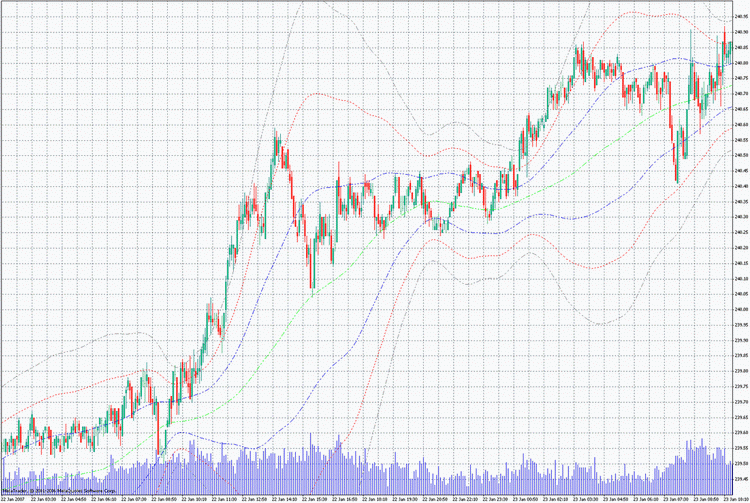Functions ParMASeries()

Below is the exemplary call for function ParMASeries() (ParMA moving with additional JMA smoothing):
```/*
Moving average ParMA calculated on parabolic
regression with bands

for the indicator to work, one should place files
JJMASeries.mqh
ParMASeries.mqh
PriceSeries.mqh
Heiken Ashi#.mq4
*/
//+------------------------------------------------------------------+
//|                                                       JParMA.mq4 |
//+------------------------------------------------------------------+
//---- drawing the indicator in the main window
#property indicator_chart_window
//---- amount of indicator buffers
#property indicator_buffers 1
//---- color of the indicator
#property indicator_color1 Red
//---- INDICATOR INPUTS
extern int MA_Period  = 8; // ParMA period
extern int Length = 3;   // smoothing depth
// parameter ranging between -100 and +100,
//it influences the transient quality;
extern int Phase  = 100;
extern int Shift  = 0;   // indicator shift along the time axis
//Choosing of prices, at which the indicator is calculated
/*(0-CLOSE, 1-OPEN, 2-HIGH, 3-LOW, 4-MEDIAN, 5-TYPICAL, 6-WEIGHTED,
7-Heiken Ashi Close, 8-SIMPL, 9-TRENDFOLLOW, 10-0.5*TRENDFOLLOW,
11-Heiken Ashi High, 12-Heiken Ashi Low, 13-Heiken Ashi Open,
14-Heiken Ashi Close.) */
extern int Input_Price_Customs = 0;
//---- indicator buffers
double IndBuffer[];
//---- float point variables
double JResalt, Price, Resalt;
//+------------------------------------------------------------------+
//----+ Introducing of function JJMASeries
//----+ Introducing of function JJMASeriesResize
//----+ Introducing of function JMA_ErrDescr
#include <JJMASeries.mqh>
//+------------------------------------------------------------------+
//----+ Introducing of function ParMAMASeries
//----+ Introducing of function ParMASeriesResize
//----+ Introducing of function ParMA_ErrDescr
#include <ParMASeries.mqh>
//+------------------------------------------------------------------+
//----+ Introducing of function PriceSeries
#include <PriceSeries.mqh>
//+------------------------------------------------------------------+
//| JParMA initialization function                                   |
//+------------------------------------------------------------------+
int init()
{
//---- Setting imaging precision format for the indicator
IndicatorDigits(Digits);
//---- defining the chart drawing style
SetIndexStyle(0, DRAW_LINE);
//---- 1 indicator buffer is used for calculations
SetIndexBuffer(0, IndBuffer);
//---- horizontal shift of the indicator line
SetIndexShift (0, Shift);
//---- setting the indicator values that will not be visible in
//     the chart
SetIndexEmptyValue(0, 0.0);
//---- name for data windows and label for subwindows
IndicatorShortName ("JParMA( Length="+Length+", Phase="+Phase+", Shift="+Shift+")");
SetIndexLabel(0, "JParMA Line");
//---- setting the bar number, starting from which there will be drawn
indicator
SetIndexDrawBegin(0, MA_Period);
//----+ Resizing buffer variables of function JJMASeries,
//nJMAnumber=1(One call for function JJMASeries)
if (JJMASeriesResize(1)!=1)return(-1);
//----+ Resizing buffer variables of function ParMASeries,
//nParMAnumber=1(One call for function ParMASeries)
if (ParMASeriesResize(1)!=1)return(-1);
//---- setting alerts for nonaccepted values of external variables
return(0);
}
//+-----------------------------------------------------------------------+
//| JParMA iteration function                                             |
//+-----------------------------------------------------------------------+
int start()
{
//---- check whether the amount of bars is sufficient for calculations
if (Bars-1<MA_Period)return(0);
//----+ Introducing of integer variables and getting bars already counted
int reset,MaxBar,MaxBarP,bar,Limit,counted_bars=IndicatorCounted();
//---- check for possible errors
if (counted_bars<0)return(-1);
//---- the last counted bar must be recalculated
if (counted_bars>0) counted_bars--;
//---- defining the oldest bar number, starting from which all bars
//will be recalculated
MaxBar=Bars-1; MaxBarP=MaxBar-MA_Period;

//---- defining the oldest bar number, starting from which new bars
//will be recalculated
Limit=Bars-counted_bars-1;

//---- Indicator calculation
for (bar=Limit; bar>=0; bar--)
{
//----+
Price=PriceSeries(Input_Price_Customs,bar);
//----+ getting the initial indicator
//----+ Call for function ParMASeries numbered as 0
Resalt=ParMASeries(0,MaxBar,Limit,MA_Period,Price,bar,reset);
//----+ check for errors in the preceding operation
if(reset!=0)return(-1);
//----+ JMA smoothing of the obtained indicator,
//parameter nJMA.MaxBar is decreased by MA_Period
//----+ Call for function JJMASeries numbered as 0,
// parameters nJMA.Phase and nJMA.Length do not change on each bar
// (nJMA.din=0)
JResalt=JJMASeries(0,0,MaxBarP,Limit,Phase,Length,Resalt,bar,reset);
//----+ check for errors in the preceding operation
if(reset!=0)return(-1);
IndBuffer[bar]=JResalt;
}
//----
return(0);
}

//+-------------------------------------------------------------------+```

In all indicators, the usually applied time-series array Close[] is replaced with function PriceSeries(). Its use should not cause any problems:

`double  PriceSeries(int Input_Price_Customs, int bar)`
Parameter Input_Price_Customs can range between 0 and 14. Depending on the value of this parameter, the function returns the price value for the current chart by number of the bar used as the second parameter: 0-CLOSE, 1-OPEN, 2-HIGH, 3-LOW, 4-MEDIAN, 5-TYPICAL, 6-WEIGHTED, 7-Heiken Ashi Close, 8-SIMPL, 9-TRENDFOLLOW, 10-0. 5*TRENDFOLLOW, 11-Heiken Ashi High, 12-Heiken Ashi Low, 13-Heiken Ashi Open, 14-Heiken Ashi Close. If necessary, some other algebraical expressions can be written in the function cases to define entry prices on the basis of time-series arrays. Indicators using function PriceSeries() are very helpful in optimization and testing of Expert Advisors.

### Conclusion

In NK_library.zip, there are over a hundred of indicators written using these algorithms. These examples are more than enough to learn how to use functions described in this articles for writing of any other indicators. All indicators in the zip file with these versions of smoothing functions support Expert Advisors and work with them without fail. Exceptions are indicators named with HTF at the end. These indicators, due to specific of their calculation cannot be used with Expert Advisors! Indicators from the zip file folders should be placed in the program folder of MetaTrader 4 Client Terminal: \MetaTrader\EXPERTS\indicators. The functions themselves are in a zip file in folder INCLUDE. The entire contents of that folder should be placed in the program folder of MetaTrader 4 Client Terminal: \MetaTrader\EXPERTS\INCLUDE.

Translated from Russian by MetaQuotes Software Corp.
Original article: https://www.mql5.com/ru/articles/1450

Attached files |
NK_library.zip (1939.17 KB)
Last comments | Go to discussion (3)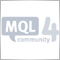| 6 Jul 2007 at 13:02

Hi Nikolay,

Thank you for a outstanding article. If you would like to use indicators with HTF at the end of the name in EA, write values to .csv and read .csv from EA.

Again, well done!!| 27 May 2008 at 00:40

Hi Nikolay,

great stuff - thanks a lot! However, when I use the functions JJMASeries () and JurXSeries () within my own indicators and then call the indicators from within an EA some strange error messages show up in the log:

2008.05.27 00:04:01    FXRangeSpotX USDCAD,Daily: JurX.Series number =0. Îøèáêà!!! Ïàðàìåòð nJurX.limit ôóíêöèè JurX.Series() áîëüøå ÷åì íåîáõîäèìî íà 1
2008.05.27 00:04:01    NNSignal USDCAD,Daily: JurXSeries number =0. Êîä îøèáêè = 4002. èíäåêñ ìàññèâà íå ñîîòâåòñòâóåò åãî ðàçìåðó
2008.05.27 00:04:01    NNSignal USDCAD,Daily: JurXSeries number =0. Ïðè èñïîëíåíèè ôóíêöèè JurXSeries() ïðîèçîøëà îøèáêà!!!
2008.05.27 00:01:47    FXRangeSpotX GBPUSD,H1: JurX.Series number =0. Îøèáêà!!! Ïàðàìåòð nJurX.limit ôóíêöèè JurX.Series() áîëüøå ÷åì íåîáõîäèìî íà 1
2008.05.27 00:01:47    NNSignal GBPUSD,H1: JurXSeries number =0. Êîä îøèáêè = 4002. èíäåêñ ìàññèâà íå ñîîòâåòñòâóåò åãî ðàçìåðó
2008.05.27 00:01:47    NNSignal GBPUSD,H1: JurXSeries number =0. Ïðè èñïîëíåíèè ôóíêöèè JurXSeries() ïðîèçîøëà îøèáêà!!!

I have followed your instructions exactly but cannot get rid of the error messages... Key question is: is there a bug in the functions or am I doing something wrong. Here is the indicator code  that causes  the problem when called from an EA:

#property indicator_separate_window
#property indicator_minimum 0
#property indicator_maximum 1
#property indicator_buffers 2
#property indicator_color1 Red
#property indicator_color2 Yellow

//---- input parameters

extern int IndexPeriod = 10;
extern int Length_0 = 5;
extern int Length_1 = 10;

extern int Input_Price_Customs = 0;

#include <SmoothAlg.mqh>

//---- buffers
double Buffer1[], Buffer2[];
//+------------------------------------------------------------------+
//| Custom indicator initialization function                         |
//+------------------------------------------------------------------+
int init()
{
string name = "FXRangeSpotX";
IndicatorShortName(name);
IndicatorBuffers(2);
//---- indicators
SetIndexStyle(0,DRAW_LINE);
SetIndexBuffer(0,Buffer1);
SetIndexShift(0, 0);
SetIndexEmptyValue(0, 0.0);

SetIndexStyle(1, DRAW_LINE);
SetIndexBuffer(1,Buffer2);
SetIndexShift(1, 0);
SetIndexEmptyValue(1, 0.0);

SetIndexLabel(0,name);
SetIndexDrawBegin(0,IndexPeriod);

JurXSeriesResize(1);

//----
return(0);
}
//+------------------------------------------------------------------+
//| Custom indicator deinitialization function                       |
//+------------------------------------------------------------------+
int deinit()
{
//----

//----
return(0);
}
//+------------------------------------------------------------------+
//| Custom indicator iteration function                              |
//+------------------------------------------------------------------+
int start()
{
int    i, limit, counted_bars;
double bulls, bears;
double dMA;

counted_bars =IndicatorCounted();
/*
Print("Bars: " + Bars + "; Counted: " + counted_bars);
Print("Time of Bars: " + TimeToStr(Time));
Print("Time of Bars[Bars-1]: " + TimeToStr(Time[Bars-1]));
*/

//---- check for possible errors
if(counted_bars<0) return(-1);
//---- the last counted bar will be recounted
if(counted_bars>0)
counted_bars--;

limit=Bars-counted_bars;

if (Bars <= IndexPeriod) { //set index buffer to 0
for (i = 0; i < limit; i++) {
Buffer1[i] = 0.0;
Buffer2[i] = 0.0;
}
return (0);
}

for (i = 0; i < limit; i++) {
Buffer1[i] = iSomeIndicator(NULL, 0, IndexPeriod, i) / 100.0;
Buffer2[i] = Buffer1[i];
}

smoothJurX(Buffer1, Length_0, counted_bars);
smoothJurX(Buffer2, Length_1, counted_bars);

return(0);
}| 17 Sep 2011 at 23:07

Hey Jurik,

its just lately that i started to get into your ideas. Very interesting & effective. Thanks a lot for sharing.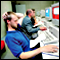What Is a Martingale?

A short description of various illusions that come up when people trade using martingale betting strategies or misuse spiking and the like approaches.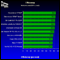Testing Visualization: Manual Trading

Testing manual strategies on history. Check how your trading algorithm works turning a deaf ear to programming niceties!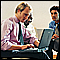MQL4 as a Trader's Tool, or The Advanced Technical Analysis

Trading is, first of all, a calculus of probabilities. The proverb about idleness being an engine for progress reveals us the reason why all those indicators and trading systems have been developed. It comes that the major of newcomers in trading study "ready-made" trading theories. But, as luck would have it, there are some more undiscovered market secrets, and tools used in analyzing of price movements exist, basically, as those unrealized technical indicators or math and stat packages. Thanks awfully to Bill Williams for his contribution to the market movements theory. Though, perhaps, it's too early to rest on oars.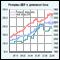Poll: Traders’ Estimate of the Mobile Terminal

Unfortunately, there are no clear projections available at this moment about the future of the mobile trading. However, there are a lot of speculations surrounding this matter. In our attempt to resolve this ambiguity we decided to conduct a survey among traders to find out their opinion about our mobile terminals. Through the efforts of this survey, we have managed to established a clear picture of what our clients currently think about the product as well as their requests and wishes in future developments of our mobile terminals.